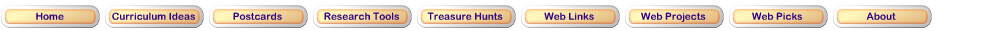Step 1: Reviewing Decimals and Fractions Before learning how to go from decimals to fractions, Review what decimals are and what they mean. Review what fractions are and what they mean.Step 2: From Decimals to FractionsYou probably use decimals and fractions more than you expect. Did you know that if you convert a decimal into a fraction it does not change its value? Try this exploration to learn how to convert decimals into fractions.Step 3: More About Converting Decimals into FractionsSandra says, "I know that 0.3 is equivalent to 3/10. I wonder if 0.03 is then equivalent to 3/100?" What do you think? How did Sandra arrive at this conclusion? Try this exploration to answer this.Step 4: Writing Larger Decimal Numbers as FractionsYou can use the same method you used in previous steps to convert decimal numbers greater than 1, such as 1.25, into fractions.Type 1.25. Start this exploration to find out how!Step 5: WorksheetConvert these decimals to fractions on this worksheet.Updated April 8, 2017
© 1996 - 2009 Linda C. Joseph